# Disdyakis triacontahedron

Disdyakis triacontahedron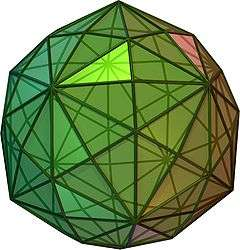animated
TypeCatalan
Conway notationmD or dbD
Coxeter diagramFace polygon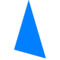scalene triangle
Faces120
Edges180
Vertices62 = 12 + 20 + 30
Face configurationV4.6.10
Symmetry groupIh, H3, [5,3], (*532)
Rotation groupI, [5,3]+, (532)
Dihedral angle164° 53' 17"
Dual polyhedrontruncated icosidodecahedron
Propertiesconvex, face-transitive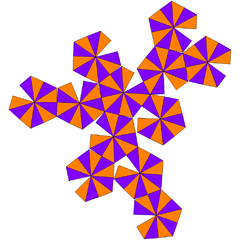Net

In geometry, a disdyakis triacontahedron, hexakis icosahedron or kisrhombic triacontahedron is a Catalan solid with 120 faces and the dual to the Archimedean truncated icosidodecahedron. As such it is face uniform but with irregular face polygons. It looks a bit like an inflated rhombic triacontahedron—if one replaces each face of the rhombic triacontahedron with a single vertex and four triangles in a regular fashion one ends up with a disdyakis triacontahedron. That is, the disdyakis triacontahedron is the Kleetope of the rhombic triacontahedron. It also has the most faces among the Archimedean and Catalan solids, with the snub dodecahedron, with 92 faces, in second place.

If the bipyramids and the trapezohedra are excluded, the disdyakis triacontahedron has the most faces of any other strictly convex polyhedron where every face of the polyhedron has the same shape.

## Symmetry

The edges of the polyhedron projected onto a sphere form 15 great circles, and represent all 15 mirror planes of reflective Ih icosahedral symmetry, as shown in this image. Combining pairs of light and dark triangles define the fundamental domains of the nonreflective (I) icosahedral symmetry. The edges of a compound of five octahedra also represent the 10 mirror planes of icosahedral symmetry.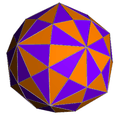Disdyakis triacontahedron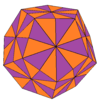Dodecahedron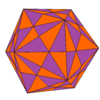Icosahedron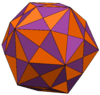Rhombic triacontahedron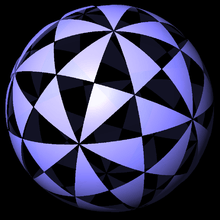Alternately colored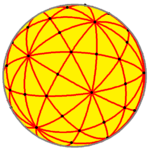Edges as great circles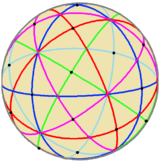compound of five octahedra

## Orthogonal projections

The disdyakis triacontahedron has three types of vertices which can be centered in orthogonally projection:

Projectivesymmetry Image Dualimage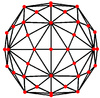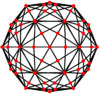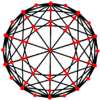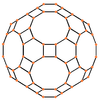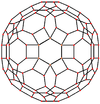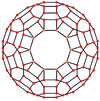## Uses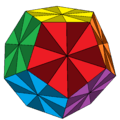Disdyakis triacontahedron on a dodecahedron

The disdyakis triacontahedron, as a regular dodecahedron with pentagons divided into 10 triangles each, is considered the "holy grail" for combination puzzles like the Rubik's cube. This unsolved problem, often called the "big chop" problem, currently has no satisfactory mechanism. It is the most significant unsolved problem in mechanical puzzles.

This shape was used to create d120 dice using 3D printing. More recently, the Dice Lab has used the Disdyakis triacontahedron to mass market an injection moulded 120 sided die. It is claimed that the d120 is the largest number of possible faces on a fair dice, aside from infinite families (such as right regular prisms, bipyramids, and trapezohedra) that would be impractical in reality due to the tendency to roll for a long time.

## Related polyhedra

It is topologically related to a polyhedra sequence defined by the face configuration V4.6.2n. This group is special for having all even number of edges per vertex and form bisecting planes through the polyhedra and infinite lines in the plane, and continuing into the hyperbolic plane for any n ≥ 7.

With an even number of faces at every vertex, these polyhedra and tilings can be shown by alternating two colors so all adjacent faces have different colors.

Each face on these domains also corresponds to the fundamental domain of a symmetry group with order 2,3,n mirrors at each triangle face vertex.

## References

• Williams, Robert (1979). The Geometrical Foundation of Natural Structure: A Source Book of Design. Dover Publications, Inc. ISBN 0-486-23729-X. (Section 3-9)
• Wenninger, Magnus (1983), Dual Models, Cambridge University Press, ISBN 978-0-521-54325-5, MR 730208 (The thirteen semiregular convex polyhedra and their duals, Page 25, Disdyakistriacontahedron )
• The Symmetries of Things 2008, John H. Conway, Heidi Burgiel, Chaim Goodman-Strass, ISBN 978-1-56881-220-5 (Chapter 21, Naming the Archimedean and Catalan polyhedra and tilings, page 285, kisRhombic triacontahedron )